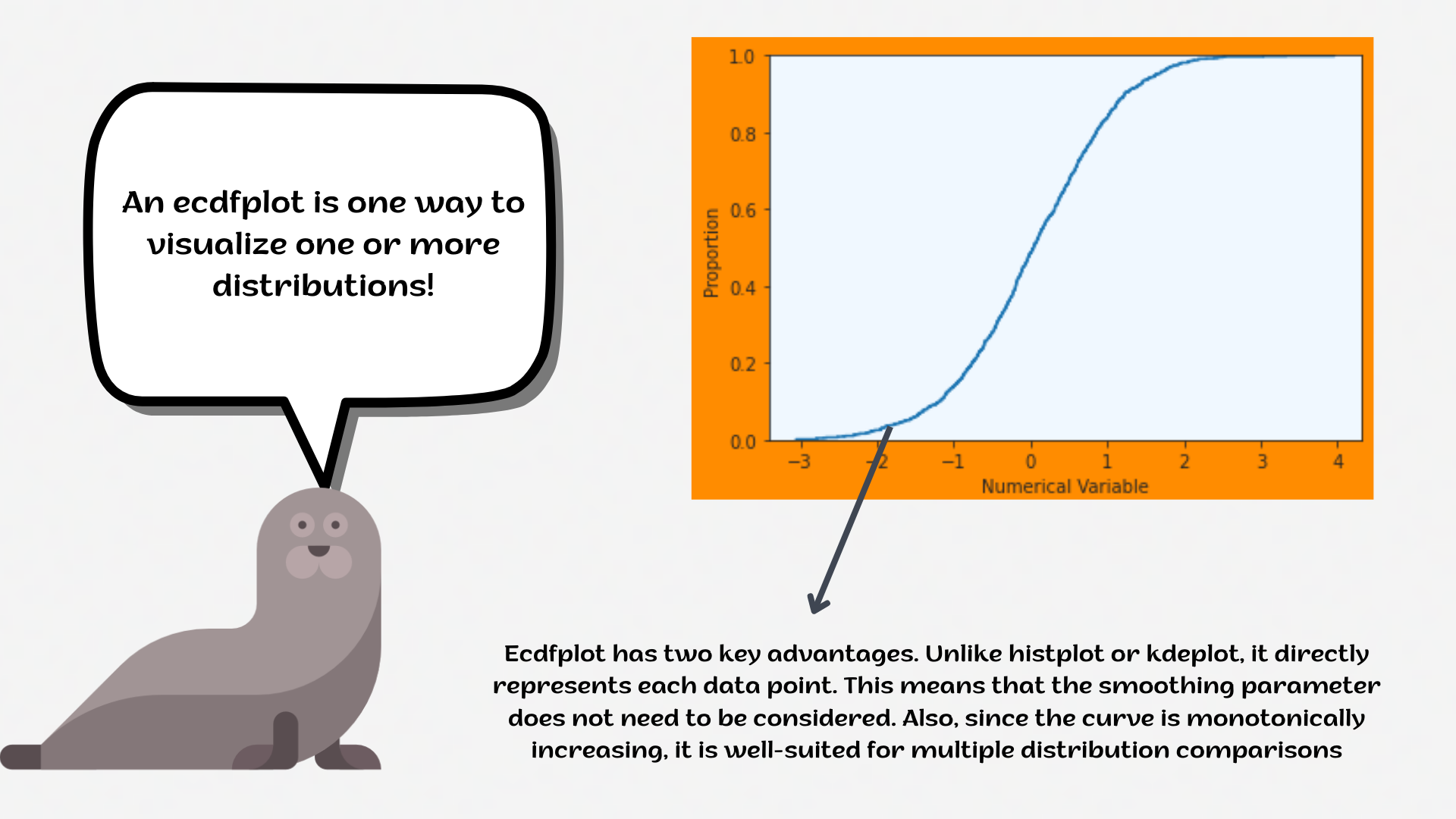Course Content

# Deep Dive into the seaborn Visualization

1. Light Start

2. Distributions of Data

3. Categorical Plot Types

4. Matrix Plots

5. Multi-Plot Grids

6. Regression Models

Deep Dive into the seaborn Visualization

##EcdfplotAn `ecdfplot` represents the proportion or count of observations falling below each unique value in a dataset. Compared to a histogram or density plot, it has the advantage that each observation is visualized directly, meaning that no binning or smoothing parameters need to be adjusted.1. Create the `ecdfplot` using the `seaborn` library:
• Set the `x` parameter equals the `bill_length_mm`;
• Set the `hue` parameter equals the `'island'`;
• Add the `complementary` parameter;
• Set the `stat` parameter equals the `'count'`;
• Set the palette equals the `'mako'`;